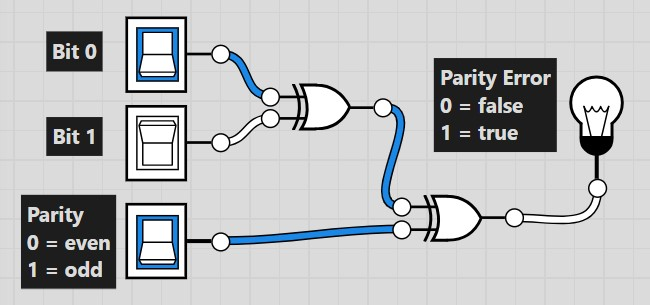On Ecampus under the "Submit Homework" menu option, submit a jpg or png image of your logic circuit and the .cpp source code file for your program.

1. In data communications, parity is sometimes used to detect transmission errors.  Assume for each byte transmitted, an extra parity bit is transmitted that is set to whether the byte has and even or odd number of 1's.  Using even parity, if the number of 1's is even, then the parity bit is set to 0.  If the number of 1's is odd, then the parity bit is set to 1.

 Transmitted Byte Count of 1's Parity Bit 11001100 4 0 11101101 6 0 10001100 3 1 10101101 5 1
If the parity bit does not match the number of 1's in the byte, then there is an error.

 Transmitted Byte Parity Bit Error 11001100 0 false 11101101 1 true 10001100 1 false 10101101 0 true

Use a tool for designing and simulating logic gates such as Logisim or logic.ly, create a working circuit that outputs true (1) or false (0) on whether a byte (8 bits) matches its parity bit.  Label the inputs and outputs appropriately.  Below is an example circuit with a 2-bit input.2
. Using C++, write a program that converts a decimal number from 0-255 to binary and hexadecimal.  Code the algorithm yourself - do not use external libraries that provide the conversions automatically.  Add leading zeroes so the answer is one byte.

Example output
This program converts an integer to binary and hex.

Enter an integer: 205
Binary: 11001101
Hex:    CD

Enter an integer: 14
Binary: 00001110
Hex:    0E